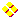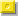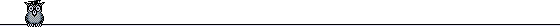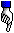HOME plateWON | World!OfNumbersJohn Abreu's Palindrome PuzzleAcross Down
To give you a helping hand in solving this puzzle
feel free to use this JavaScript fill-in board.
Press the button to extract and study
the Down/Across numbers.

Enjoy the puzzle!In order to have my puzzle well stated let us define the concept of  Down/Across Addition.
For that we use the following example :
 7 8 9 | 4 4 5 6 | 7 + 1 2 3 | 7 ———— 1 3 6 8 Given the numbers 789, 456 and 123, the Down/Across Addition is the couple [ 1368 , 774 ]. The first member of the couple 1368 being the ordinary sum of the three numberswritten from top to bottom in the same order as they were given. To obtain the second member of the couple 774 rotate the paper 90 degrees to the right and then add. In the example on the left the result is to be read from bottom to top.

P U ZZ L E

 [A]Enter the integers from 1 through 9 in a (3 x 3) array so that the Down/Across Addition gives : [ A palindrome , The square of a palindrome ] [B]Enter the integers from 1 through 9 in a (3 x 3) array so that the Down/Across Addition gives : [ A palindrome , Twice the palindrome ] [C]Enter the integers from 1 through 9 in a (3 x 3) array so that the Down/Across Addition gives : [ A palindrome , A palindrome ] In each of the puzzles there could be more than one solution. I hope you enjoy these puzzles and... by the way, do not forget to use all integers from 1 through 9 just once. If you don't follow the rules with the board an alert message will remind them to you !

John Abreu (email) is the author of the above puzzle.

Various Sources

Nine Digits

I've got this from rec.puzzles

There is a 9-digit number in which the digits 1 through 9 appear exactly once.
If you only take the first N digits from the left, the number you're left with is
divisible by that same value N. What is this unique number ?
It's up to you to reveal the solution ( hint: the Source of the Solution can be Viewed... )

If you like such puzzles please try to contribute to The Nine Digits Page

This problem was found by Stan Wagon in Leningrad Mathematical Olympiads, 1987-1991,
MathPro Press, pp. 7, 62 and was sent to me by John Abreu.

What is the greatest integer so that each of its interior digits is less than the average of its neighbors ?

The answer is surprise surprise... a palindrome !   96433469

```

```

[TOP OF PAGE]

Patrick De Geest - Belgium- Short Bio - Some Pictures